# Acceleration or equilibrium practice quizPage 1

#### WATCH ALL SLIDES

Slide 1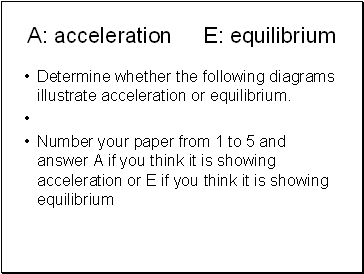## A: acceleration E: equilibrium

Determine whether the following diagrams illustrate acceleration or equilibrium.

Number your paper from 1 to 5 and answer A if you think it is showing acceleration or E if you think it is showing equilibrium

Slide 2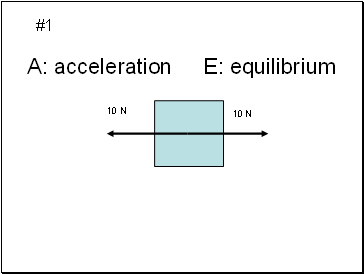A: acceleration E: equilibrium

10 N

10 N

#1

Slide 3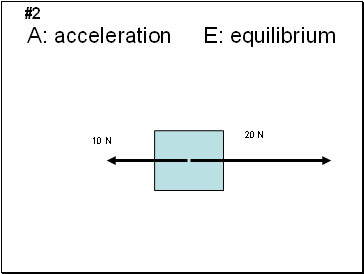A: acceleration E: equilibrium

10 N

20 N

#2

Slide 4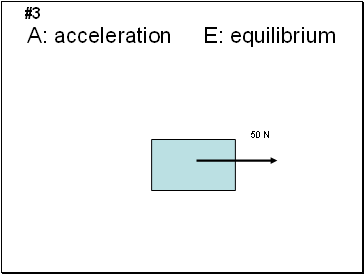A: acceleration E: equilibrium

50 N

#3

Slide 5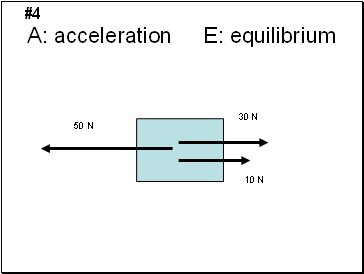A: acceleration E: equilibrium

50 N

30 N

10 N

#4

Slide 6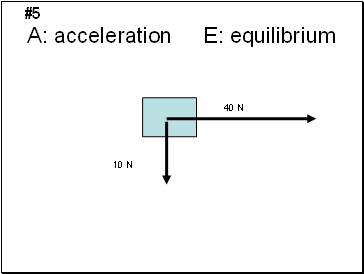A: acceleration E: equilibrium

10 N

40 N

#5

Slide 7A: acceleration B: equilibrium

1

20 N

20 N

10 N

10 N

#6

Slide 8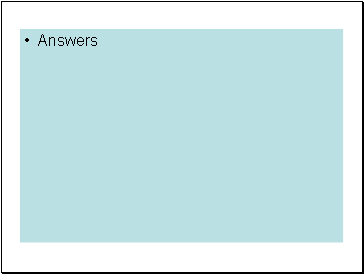Slide 9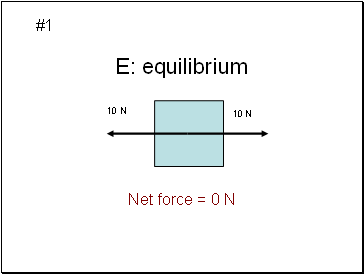E: equilibrium

10 N

10 N

#1

Net force = 0 N

Slide 10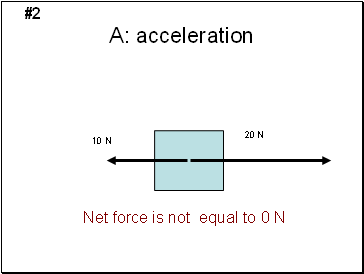A: acceleration

10 N

20 N

#2

Net force is not equal to 0 N

Slide 11A: acceleration

50 N

#3

Net force is not equal to 0 N

Slide 12A: acceleration

50 N

30 N

10 N

#4

Net force is not equal to 0 N

Slide 13A: acceleration

10 N

40 N

#5

Net force is not equal to 0 N

Slide 14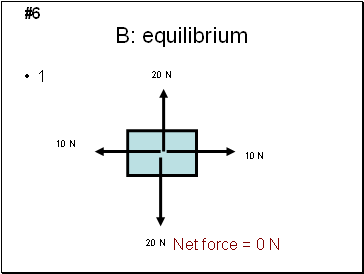B: equilibrium

1

20 N

20 N

10 N

10 N

#6

Net force = 0 N